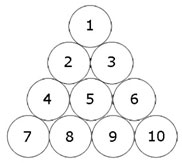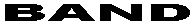# Missing Letters

Find the missing letters which complete the group.

B, C, D, E, ?, P, T, ?

# Number Puzzle

Make 100 using six 9. You can use addition, division and fraction.

# July Equals To…

If March = 43 and May = 39, what does July equals to?

# Quick and Easy Puzzle

Find the values of  a and b:

12 x a + b = 98

123 x a + b+1 = 987

1234 x a + b+2 = 9876

12345 x a + b+3 = 98765

123456 x a + b+4 = 987654

1234567 x a + b+5 = 9876543

12345678 x a + b+6 = 98765432

123456789 x a + b+7 = 987654321

# Invert The Triangle With Three Moves

By changing the position of only three circles, rearrange the triangle upside down so that the base is on top and the point is at the bottom.# Solve the Rebus Puzzles

1) estimate
don’t

2)

SAND
A     N
N     A
DNAS

3)

arrest
U R

4)  DOWN DOWN EARTH

5)